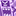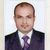# QlikView App Dev

Discussion Board for collaboration related to QlikView App Development.

Announcements
Join us at the Cloud Data and Analytics Tour! REGISTER TODAY
cancel
Showing results for
Search instead for
Did you mean:Contributor III

## Aggregating data using preceding load.

Hi,

Using my first set of QVDs I want to tranform some data and load this at an aggregated level.

My first set of QVDs is at a very low granularity, I have few flags derived at that granularity but would need the data only for flag =1 .

Eg:

QVD at detailed level

Month   Account Num  Flag Amount

1             xx                      1            10

1             yy                      0             20

Summary QVD (where flag =1 )

Month            Amount

1                         10

Can I do a preceding load where I have only the data for flag = 1?

Thanks.

1 Solution

Accepted SolutionsMVP

You can also do it like

LOAD Month, Sum(If(Flag=1,Amount)) as AmountFlag1, Sum(If(Flag=0,Amount)) as AmountFlag0

FROM YourQVD (qvd)

GROUP BY Month;

6 RepliesMVP

May be like this

LOAD Month,

Amount

Where Flag = 1;

LOAD Month,

AccountNum,

Flag,

Amount

FROM ...MVP

Try

LOAD Month, Sum(Amount) as Amount

FROM YourQVD (qvd)

WHERE Flag = 1

GROUP BY Month;MVP

In summary qvd why not

Noconcatenate

Load * Resident DetailQvd Where Flag=1;Contributor III
Author

Thank you all.

I realised that there are more measures, some measures I need to bring it for both flag 0 and 1 and some with flag 1.

By using the where flag = 1 wouldnt work in this case.

Whats the best approach at the script level?

Is it a case of doing 2 loads one for flag = 1 and one without and concatenate?MVP

You can also do it like

LOAD Month, Sum(If(Flag=1,Amount)) as AmountFlag1, Sum(If(Flag=0,Amount)) as AmountFlag0

FROM YourQVD (qvd)

GROUP BY Month;Contributor III
Author

thanks so much Stefan and others.